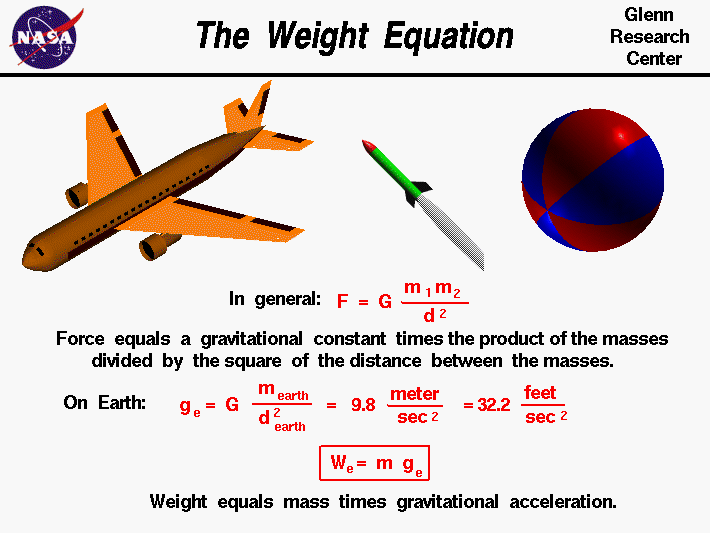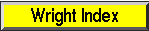+ Text Only Site
+ Non-Flash Version
+ Contact GlennThis page is intended for college, high school, or middle school students. For younger students, a simpler explanation of the information on this page is available on the Kids Page.Weight is the force generated by the gravitational attraction of the earth on any object. Weight is fundamentally different from the aerodynamic forces, lift and drag. Aerodynamic forces are mechanical forces and the object has to be in physical contact with the air which generates the force. The gravitational force is a field force; the source of the force does not have to be in physical contact with the object.

The nature of the gravitational force has been studied by scientists for many years and is still being investigated by theoretical physicists. For an object the size of an airplane flying near the earth, the descriptions given three hundred years ago by Sir Isaac Newton work quite well. Newton published his theory of gravitation with his laws of motion in 1686. The gravitational force, F, between two particles equals a universal constant, G, times the product of the mass of the particles, m1 and m2, divided by the square of the distance, d, between the particles.

F = G * m1 * m2 / d^2

If you have a lot of particles acting on a single particle, you have to add up the contribution of all the individual particles. For objects near the earth, the sum of the mass of all the particles is simply the mass of the earth and the distance is then measured from the center of the earth. On the surface of the earth the distance is about 4000 miles. Scientists have combined the universal gravitational constant, the mass of the earth, and the square of the radius of the earth to form the gravitational acceleration, g . On the surface of the earth, its value is 9.8 meters per square second or 32.2 feet per square second.

g = G * m earth / (d earth)^2

The weight W, or gravitational force, is then just the mass of an object times the gravitational acceleration.

W = m * g

Since the gravitational constant (g) depends on the square of the distance from the center of the earth, the weight of an object decreases with altitude.

Let's do a test problem to see how much the weight of an airplane changes with altitude. If an airplane is flying at 35000 feet (about 7 miles) the distance to the center of the earth is about 4007 miles. We can calculate the ratio of the gravitational constant to the value at the surface of the earth as the square of (4000/4007) which equals .9983*.9983 = .9965. If the airplane weighs 10000 pounds on the surface of the earth, it weighs 9965 pounds at 35000 feet; it has lost 35 pounds, a very small amount compared to 10000 pounds.

Let's do another problem and compute the weight of the Space Shuttle in low earth orbit. On the ground, the orbiter weighs about 250,000 pounds. In orbit, the shuttle is about 200 miles above the surface of the earth. As before, the gravitational constant ratio is the square of (4000/4200) which equals .9523*.9523 = .907. On orbit, the shuttle weighs 250,000 * .907 = 226,757 pounds. Notice: the weight is not zero. The shuttle is not weightless in orbit. "Weightlessness" is caused by the speed of the shuttle in orbit. The shuttle is pulled towards the earth because of gravity. But the high orbital speed, tangent to the surface of the earth, causes the fall towards the surface to be exactly matched by the curvature of the earth away from the shuttle. In essence, the shuttle is constantly falling all around the earth.

You can view a short movie of "Orville and Wilbur Wright" discussing the weight force and how it affected the flight of their aircraft. The movie file can be saved to your computer and viewed as a Podcast on your podcast player.

Activities:Guided Tours+ Inspector General Hotline + Equal Employment Opportunity Data Posted Pursuant to the No Fear Act + Budgets, Strategic Plans and Accountability Reports + Freedom of Information Act + The President's Management Agenda + NASA Privacy Statement, Disclaimer, and Accessibility CertificationEditor: Nancy Hall NASA Official: Nancy Hall Last Updated: May 13 2021 + Contact Glenn